## 5.2.5 Quantization of the ISP coefficients

26.1903GPPAdaptive Multi-Rate - Wideband (AMR-WB) speech codecSpeech codec speech processing functionsTranscoding functionsTS

The LP filter coefficients are quantized using the ISP representation in the frequency domain; that is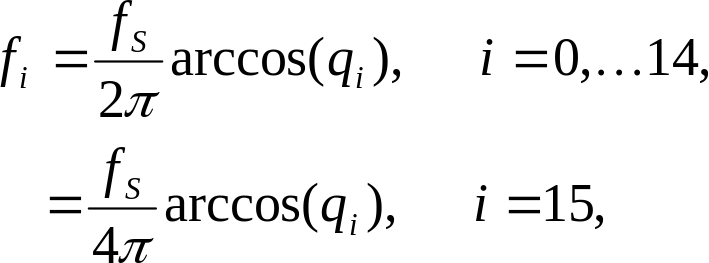( 21 )

where fi are the ISFs in Hz [0,6400] and fs=12800 is the sampling frequency. The ISF vector is given by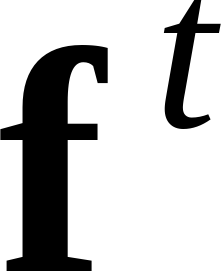= [f0f1,…,f15], with t denoting transpose.

A 1st order MA prediction is applied, and the residual ISF vector is quantified using a combination of split vector quantization (SVQ) and multi-stage vector quantization (MSVQ). The prediction and quantization are performed as follows. Let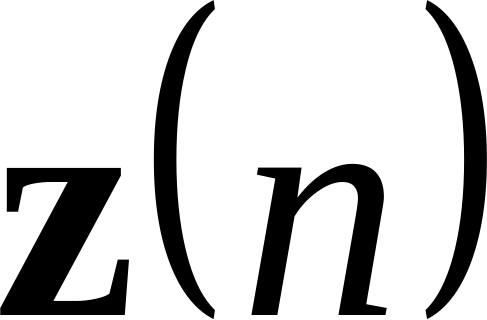denote the mean‑removed ISF vector at frame. The prediction residual vector r(n) is given by: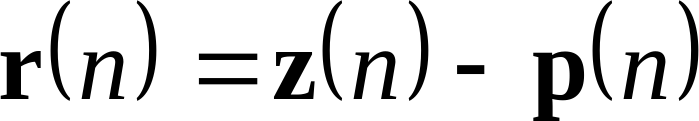( 22 )

where p(n) is the predicted LSF vector at frame n. First order moving‑average (MA) prediction is used where: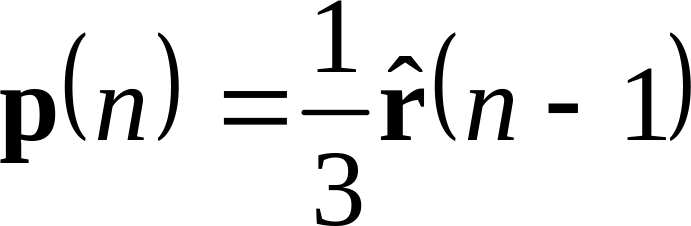, ( 23 )

where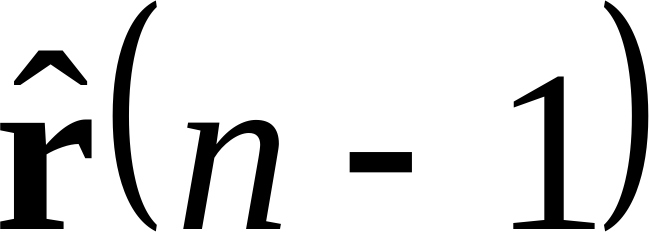is the quantized residual vector at the past frame.

The ISF residual vectoris quantized using split-multistage vector quantization S-MSVQ. The vector is split into 2 subvectors r1(n) and r2(n) of dimensions 9 and 7, respectively. The 2 subvectors are quantized in two stages. In the first stage r1(n) is quantized with 8 bits and r2(n) with 8 bits.

For 8.85 ,12.65, 14.25, 15.85, 18.25, 19.85, 23.05 or 23.85 kbit/s modes, the quantization error vectors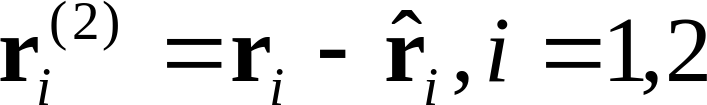are split in the next stage into 3 and 2 subvectors, respectively. The subvectors are quantized using the bit-rates described in Table 2.

Table 2. Quantization of ISP vector for the 8.85 ,12.65, 14.25, 15.85, 18.25, 19.85, 23.05 or 23.85 kbit/s modes

 1. UNQUANTIZED 16-ELEMENT-LONG ISP VECTOR 2. STAGE 1 () 8 bits 2. STAGE 1 () 8 bits 3. STAGE 2 (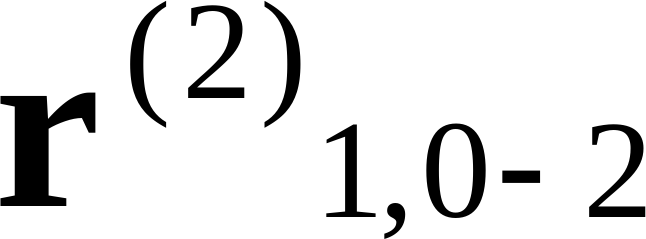) 6 bits 3. STAGE 2 (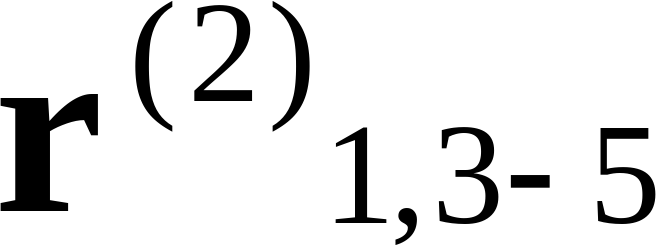) 7 bits 3. STAGE 2 (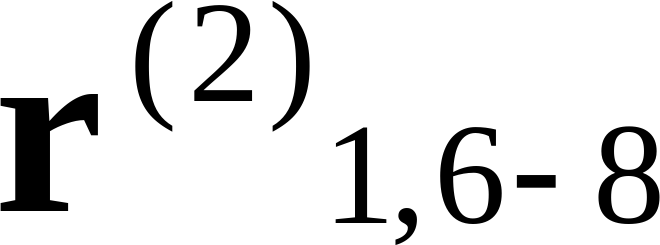) 7 bits 3. STAGE 2 (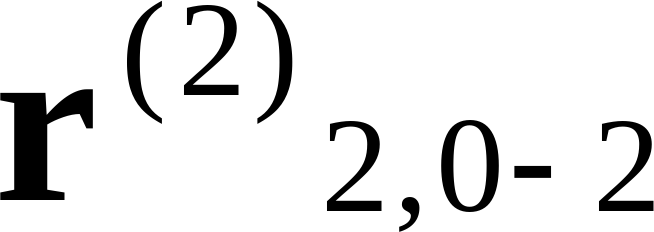) 5 bits 3. STAGE 2 (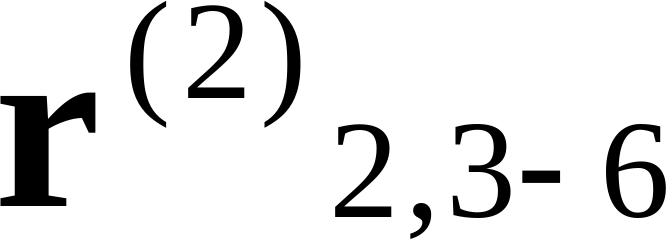) 5 bits

For 6.60 kbit/s mode, the quantization error vectors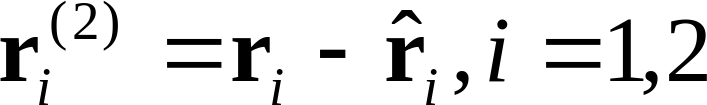are split in the next stage into 2 and 1 subvectors, respectively. The subvectors are quantized using the bit-rates described in Table 3.

Table 3. Quantization of ISP vector for the 6.60 kbit/s mode

 1. UNQUANTIZED 16-ELEMENT-LONG ISP VECTOR 2. STAGE 1 () 8 bits 2. STAGE 1 () 8 bits 3. STAGE 2 (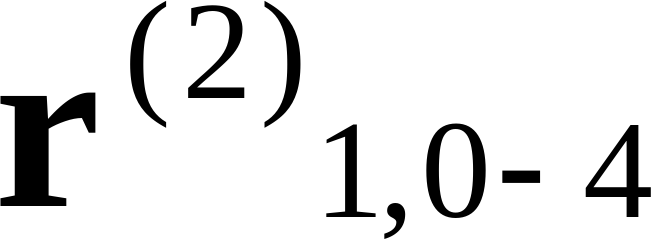) 7 bits 3. STAGE 2 (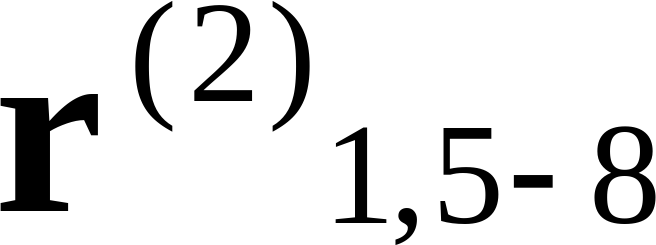) 7 bits 3. STAGE 2 (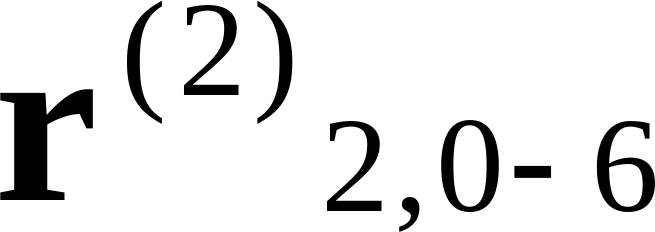) 6 bits

A squared error ISP distortion measure is used in the quantization process. In general, for an input ISP or error residual subvector ri,i=1,2 and a quantized vector at index k,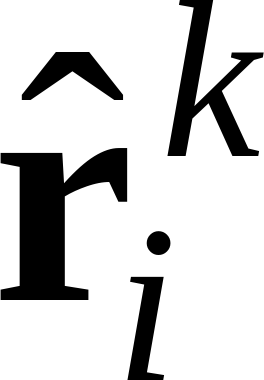, the quantization is performed by finding the index k which minimizes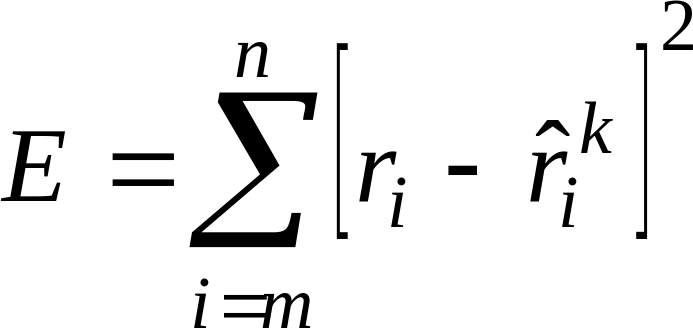, ( 24 )

where m and n are the first and last elements of the subvector.